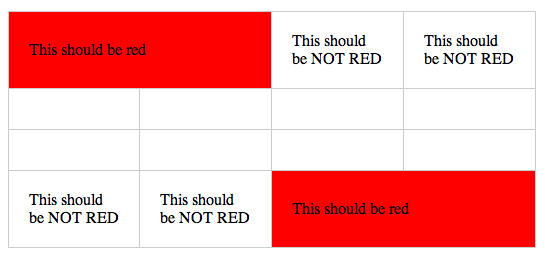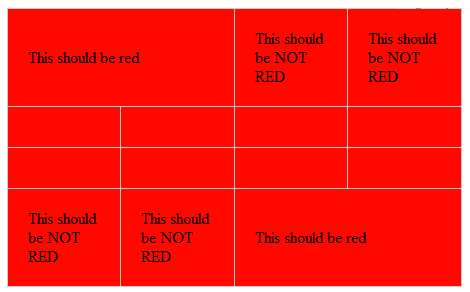IE8 中关于 colspan 属性的 bug

IE8 认为所有的表格单元格都具有 colfont 属性。因此如果需要单独针对具有 colfont 属性的单元格设置样式，就有点棘手了。

`td[colfont],th[colfont] {/* 注意：不管单元格是否有 colfont 属性，在 IE8 中该样式一律是生效的even if they don't have a colfont */background: red;}``th[colfont],th[colfont="1"],th[colfont*="1"],th[colfont^="1"],th[colfont\$="1"],td[colfont],td[colfont="1"],td[colfont*="1"],td[colfont^="1"],td[colfont\$="1"] {/* IE8 中所有可能出现问题的情况 */}`

`th[colfont*="0"],th[colfont*="2"],th[colfont*="3"],th[colfont*="4"],th[colfont*="5"],th[colfont*="6"],th[colfont*="7"],th[colfont*="8"],th[colfont*="9"],th[colfont*="11"] {/* styles */}td[colfont*="0"],td[colfont*="2"],td[colfont*="3"],td[colfont*="4"],td[colfont*="5"],td[colfont*="6"],td[colfont*="7"],td[colfont*="8"],td[colfont*="9"],td[colfont*="11"] {/* styles */}`------分隔线----------------------------

------分隔线----------------------------15粉丝
7关注